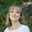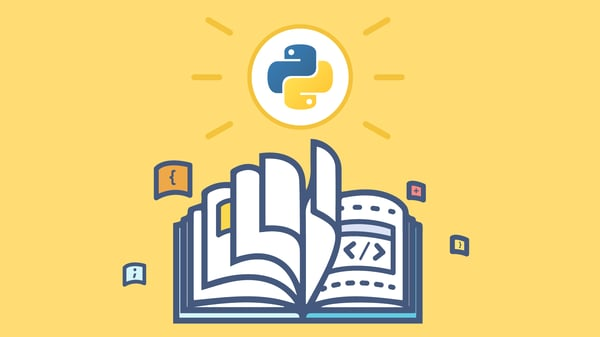Home/Blog/Languages/Level up your Python skills with these 6 challenges

# Level up your Python skills with these 6 challenges

Oct 28, 2019 - 7 min readAmanda FawcettThe best way to learn Python is to practice, practice, practice.

That’s why we’re sharing this article: so you can test out your basic Python skills with these six challenges.

These exercises are useful for everyone, especially if you’re a beginner with basic knowledge of Python concepts.

The solutions are offered on the tab to the right of the challenge. There is a hint for each challenge if you get stuck.

Here are the questions we’ll explore today:

## Challenge #1: Test your basic skills

Your challenge is to write a Python program that prints the following messages. Each of the three inputs should print on a new line.

Hello World
Let's Learn Python

Try it out yourself before looking at the solution!

# write your code here

### Explanation of Challenge #1

First, we use the print statement, which will display the result. We type our text within the ( ) . We have to surround our text with “quotations marks” since it is a string.

To start our second line of text, we hit enter and repeat this process on line 2 and 3 because each call to print will move the output to a new line. If you’re dealing with numerical input, you won’t need to add quotation marks.

Quick tip: For strings, you can use either double quotation marks (" ") or single (' '). Double quotation marks should be used if your sentence or word makes use of an apostrophe. In our example, "Let's learn Python", if you were to use single quotes you’d receive an error.

## Challenge #2: Test your knowledge of data types and variables

Your challenge is to write a Python program that calculates and prints the gravitational force between the Earth and the Sun using the grav_force variable.

The formula for gravitational force is as follows:

$F = \frac{GMm}{r^2}$

F is the total gravitation force. G is the gravitational constant. M and m are the masses to be compared, and r is the distance between those masses.

The values you will need to calculate the gravitation force of the Earth and Sun are offered below.

• G = 6.67 x 10-11
• MSun = 2.0 x 1030
• mEarth = 6.0 x 1024
• r = 1.5 x 1011

Try it out yourself before looking at the answer.

# write your code here

### Explanation of Challenge #2

This challenge is actually simpler than it seems! Don’t let the big numbers concern you. We use our arithmetic operators to perform all the operations. The * operator multiplies variables, the \ operator divides variables, and the ** performs an exponent operation.

First, we define each of our values (G, M, m, r). This stores them in the grav_force variable. We then define the grav_force equation using Python syntax and ask the program to print the answer. The parentheses are actually optional: we just used them to separate the top and bottom of the fraction.

## Challenge #3: Test your knowledge of conditional statements

Your challenge is to write a Python program that calculates the discounted price of an object using the if-elif-else statement.

• If the price is 300 or above, it will be discounted by 30%
• If the price falls between 200 and 300, it will be discounted by 20%
• If the price falls between 100 and 200, it will be discounted by 10%
• If the price is less than 100, it will be discounted by 5%
• There is no discount for negative prices

price = 350


Try it out yourself before looking at the answer.

# write your code here

### Explanation of Challenge #3

To complete this challenge, we first define the price and then all our conditions using Python syntax. You only need to specify the lowest number of each condition since the higher number is accounted for in the previous condition. We calculate a discounted price with price * (1 - discount).

We then ask the program to print our discounted price.

## Challenge #4: Test your knowledge of functions.

Your challenge is to write a Python program using the rep_cat function, which takes two integers (x and y) and converts them into strings. The string value of x should repeat 8 times, and the string value of y should repeat 5 times. The y string must then be concatenated to the x string and returned as a single piece of data.

x = 7
y = 2


Try it out yourself before looking at the solution.

# write your code here

### Explanation of Challenge #4

First, we convert the integers to strings using the str( ) method. We then can use the * operator to replicate the strings the required number of times. To link the strings together, we used the + operator, and finally, that new string is returned using the return statement.

We use print to display the final statement.

## Challenge #5: Test your knowledge of loops

Your challenge is to write the Fibonacci function so that is takes a positive number, n, and returns the n-th number in the Fibonacci sequence using loops.

The Fibonacci sequence is a famous mathematical formula where each number in the sequence is the sum of the two numbers before it. The sequence goes as follows: 0, 1, 1, 2, 3, 5, 8, 13, 21, 34, etc.

n = 10


Try it out yourself before looking at the solution.

# write your code here

### Explanation of Challenge #5

This challenge requires conditional statements. If n is less than 1, it will return -1, and if n is 1 or 2, it returns the first or second value. Note that the first two values are set, as they will always be fixed with this sequence.

We used a while loop to complete the challenge, but a for loop would also get the same result. We use the count variable and start at 3 because we already know the first two values. The two previous terms, second and fib_n become first and second with every iteration of the code.

We then ask the program to print our specified value, n.

## Challenge #6: Test your knowledge of data structures

Write a Python program that separates the highs and lows of a list of numbers (num_list) then returns a list of the number of lows and highs, in that order. You will use the count_low_high() function. These are your parameters.

• If a number is more than 50 or divisible by 3, it is considered high
• If these conditions are not met, the number is considered low
• If the list is empty, it will return none

num_list = [77, 9, 95, 2, 51, 29, 12, 136]


Try it out yourself before looking at the solution.

# write your code here

### Explanation to Challenge #6

To complete this challenge, you need to use the filter ( ) function, which filters the high numbers into one list and the low numbers into another. We set the parameters for sorting. We can then use the len function, which counts the elements in both lists. It isn’t necessary to use the lambdas, but it simplifies the code. The lambdas keyword makes a shortcut to declare functions.

We then input our num_list and ask the program to print our new list.

## What to learn next

Well done! You completed all six Python challenges! You’re now a more seasoned and practiced developer. Don’t be discouraged if you got stuck on some of the challenges. The best way to learn is to identify places for improvement and study.

Remember that everyone learns at their own pace and style, so try not to compare your progress to others.

If you want to keep learning, check out our free Learn Python from Scratch course that walks you through all of these concepts in more detail.

Happy learning!WRITTEN BYAmanda Fawcett

Join a community of 1.7 million readers. Enjoy a FREE, weekly newsletter rounding up Educative's most popular learning resources, coding tips, and career advice.

Learn in-demand tech skills in half the time